# 0.1 Matrix concepts -- multiplying matrices

 Page 1 / 1
This module covers multiplication of matrices.

## Multiplying a row matrix by a column matrix

A “row matrix” means a matrix with only one row. A “column matrix” means a matrix with only one column. When a row matrix has the same number of elements as a column matrix, they can be multiplied. So the following is a perfectly legal matrix multiplication problem:

$\left[\begin{array}{cccc}1& 2& 3& 4\end{array}\right]x\left[\begin{array}{c}10\\ 20\\ 30\\ 40\end{array}\right]$

These two matrices could not be added, of course, since their dimensions are different, but they can be multiplied. Here’s how you do it. You multiply the first (left-most) item in the row, by the first (top) item in the column. Then you do the same for the second items, and the third items, and so on. Finally, you add all these products to produce the final number.

A couple of my students (Nakisa Asefnia and Laura Parks) came up with an ingenious trick for visualizing this process. Think of the row as a dump truck, backing up to the column dumpster. When the row dumps its load, the numbers line up with the corresponding numbers in the column, like so:

So, without the trucks and dumpsters, we express the result—a row matrix, times a column matrix—like this:

$\left[\begin{array}{cccc}1& 2& 3& 4\end{array}\right]\left[\begin{array}{c}10\\ 20\\ 30\\ 40\end{array}\right]=\left[\begin{array}{c}300\end{array}\right]$

• The picture is a bit deceptive, because it might appear that you are multiplying two columns. In fact, you cannot multiply a column matrix by a column matrix . We are multiplying a row matrix by a column matrix. The picture of the row matrix “dumping down” only demonstrates which numbers to multiply.
• The answer to this problem is not a number: it is a 1-by-1 matrix.
• The multiplication can only be performed if the number of elements in each matrix is the same. (In this example, each matrix has 4 elements.)
• Order matters! We are multiplying a row matrix times a column matrix , not the other way around.

It’s important to practice a few of these, and get the hang of it, before you move on.

## Multiplying matrices in general

The general algorithm for multiplying matrices is built on the row-times-column operation discussed above. Consider the following example:

$\left[\begin{array}{ccc}1& 2& 3\\ 4& 5& 6\\ 7& 8& 9\\ \text{10}& \text{11}& \text{12}\end{array}\right]$ $\left[\begin{array}{cc}\text{10}& \text{40}\\ \text{20}& \text{50}\\ \text{30}& \text{60}\end{array}\right]$

The key to such a problem is to think of the first matrix as a list of rows (in this case, 4 rows), and the second matrix as a list of columns (in this case, 2 columns). You are going to multiply each row in the first matrix, by each column in the second matrix. In each case, you will use the “dump truck” method illustrated above.

Start at the beginning: first row, times first column.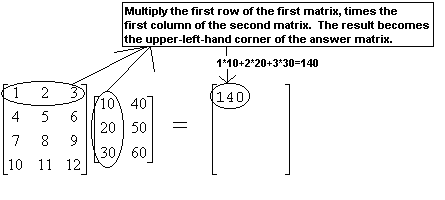Now, move down to the next row. As you do so, move down in the answer matrix as well.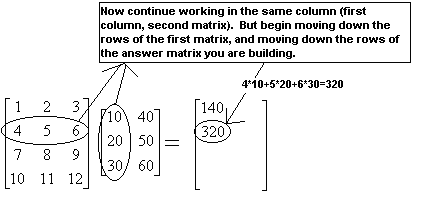Now, move down the rows in the first matrix, multiplying each one by that same column on the right. List the numbers below each other.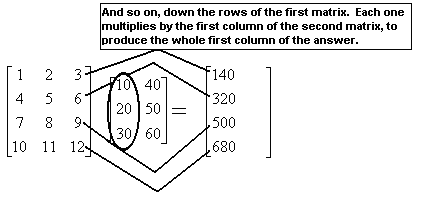The first column of the second matrix has become the first column of the answer. We now move on to the second column and repeat the entire process, starting with the first row.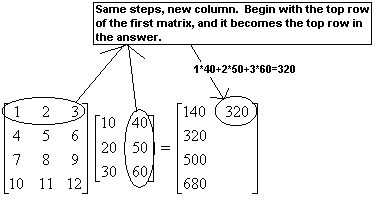And so on, working our way once again through all the rows in the first matrix.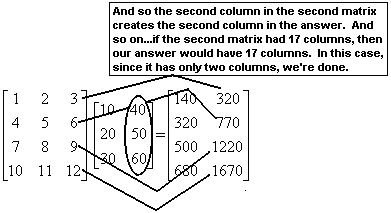We’re done. We can summarize the results of this entire operation as follows:

$\left[\begin{array}{ccc}1& 2& 3\\ 4& 5& 6\\ 7& 8& 9\\ \text{10}& \text{11}& \text{12}\end{array}\right]$ $\left[\begin{array}{cc}\text{10}& \text{40}\\ \text{20}& \text{50}\\ \text{30}& \text{60}\end{array}\right]$ $=$ $\left[\begin{array}{cc}\text{140}& \text{320}\\ \text{320}& \text{770}\\ \text{500}& \text{1220}\\ \text{680}& \text{1670}\end{array}\right]$

It’s a strange and ugly process—but everything we’re going to do in the rest of this unit builds on this, so it’s vital to be comfortable with this process. The only way to become comfortable with this process is to do it. A lot. Multiply a lot of matrices until you are confident in the steps.

Note that we could add more rows to the first matrix, and that would add more rows to the answer. We could add more columns to the second matrix, and that would add more columns to the answer. However—if we added a column to the first matrix, or added a row to the second matrix, we would have an illegal multiplication. As an example, consider what happens if we try to do this multiplication in reverse:

$\left[\begin{array}{cc}\text{10}& \text{40}\\ \text{20}& \text{50}\\ \text{30}& \text{60}\end{array}\right]$ $\left[\begin{array}{ccc}1& 2& 3\\ 4& 5& 6\\ 7& 8& 9\\ \text{10}& \text{11}& \text{12}\end{array}\right]$ Illegal multiplication

If we attempt to multiply these two matrices, we start (as always) with the first row of the first matrix, times the first column of the second matrix: $\left[\begin{array}{cc}10& 40\end{array}\right]$ $\left[\begin{array}{c}1\\ 4\\ 7\\ \text{10}\end{array}\right]$ . But this is an illegal multiplication; the items don’t line up, since there are two elements in the row and four in the column. So you cannot multiply these two matrices.

This example illustrates two vital properties of matrix multiplication.

• The number of columns in the first matrix, and the number of rows in the second matrix, must be equal. Otherwise, you cannot perform the multiplication.
• Matrix multiplication is not commutative —which is a fancy way of saying, order matters. If you reverse the order of a matrix multiplication, you may get a different answer, or you may (as in this case) get no answer at all.

How we are making nano material?
what is a peer
What is meant by 'nano scale'?
What is STMs full form?
LITNING
scanning tunneling microscope
Sahil
what is Nano technology ?
write examples of Nano molecule?
Bob
The nanotechnology is as new science, to scale nanometric
brayan
nanotechnology is the study, desing, synthesis, manipulation and application of materials and functional systems through control of matter at nanoscale
Damian
Is there any normative that regulates the use of silver nanoparticles?
what king of growth are you checking .?
Renato
What fields keep nano created devices from performing or assimulating ? Magnetic fields ? Are do they assimilate ?
why we need to study biomolecules, molecular biology in nanotechnology?
?
Kyle
yes I'm doing my masters in nanotechnology, we are being studying all these domains as well..
why?
what school?
Kyle
biomolecules are e building blocks of every organics and inorganic materials.
Joe
anyone know any internet site where one can find nanotechnology papers?
research.net
kanaga
sciencedirect big data base
Ernesto
Introduction about quantum dots in nanotechnology
what does nano mean?
nano basically means 10^(-9). nanometer is a unit to measure length.
Bharti
do you think it's worthwhile in the long term to study the effects and possibilities of nanotechnology on viral treatment?
absolutely yes
Daniel
how to know photocatalytic properties of tio2 nanoparticles...what to do now
it is a goid question and i want to know the answer as well
Maciej
Abigail
for teaching engĺish at school how nano technology help us
Anassong
How can I make nanorobot?
Lily
Do somebody tell me a best nano engineering book for beginners?
there is no specific books for beginners but there is book called principle of nanotechnology
NANO
how can I make nanorobot?
Lily
what is fullerene does it is used to make bukky balls
are you nano engineer ?
s.
fullerene is a bucky ball aka Carbon 60 molecule. It was name by the architect Fuller. He design the geodesic dome. it resembles a soccer ball.
Tarell
what is the actual application of fullerenes nowadays?
Damian
That is a great question Damian. best way to answer that question is to Google it. there are hundreds of applications for buck minister fullerenes, from medical to aerospace. you can also find plenty of research papers that will give you great detail on the potential applications of fullerenes.
Tarell
what is the Synthesis, properties,and applications of carbon nano chemistry
Mostly, they use nano carbon for electronics and for materials to be strengthened.
Virgil
is Bucky paper clear?
CYNTHIA
carbon nanotubes has various application in fuel cells membrane, current research on cancer drug,and in electronics MEMS and NEMS etc
NANO
Difference between extinct and extici spicies
Got questions? Join the online conversation and get instant answers!ByBy Gerr Zen